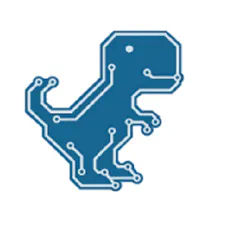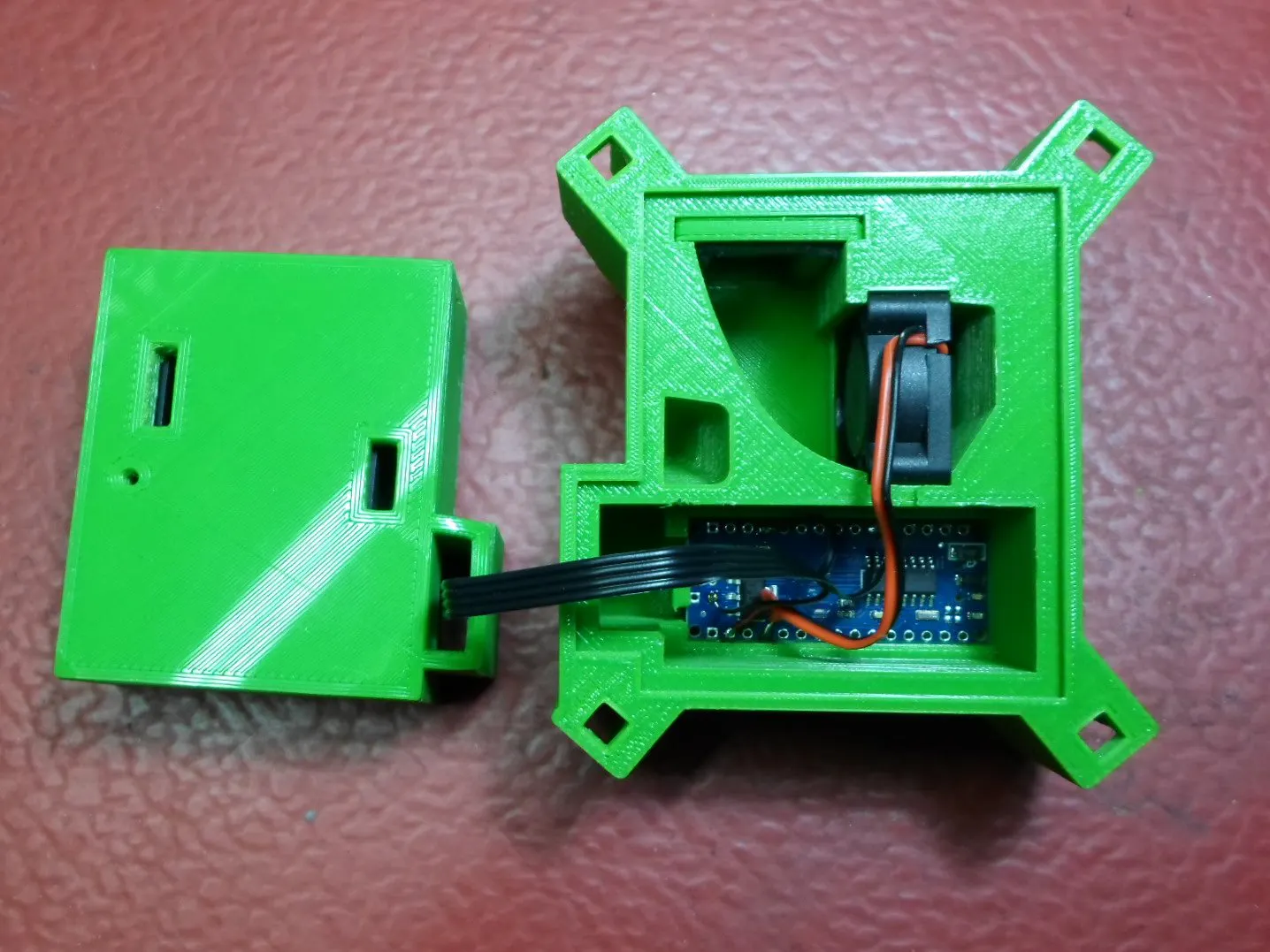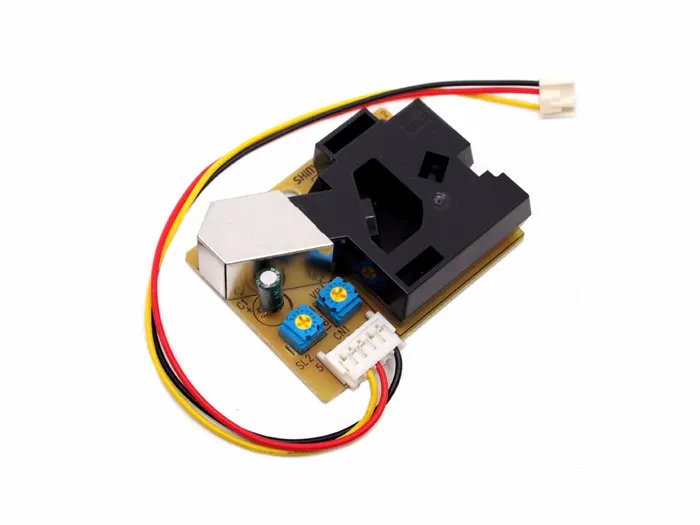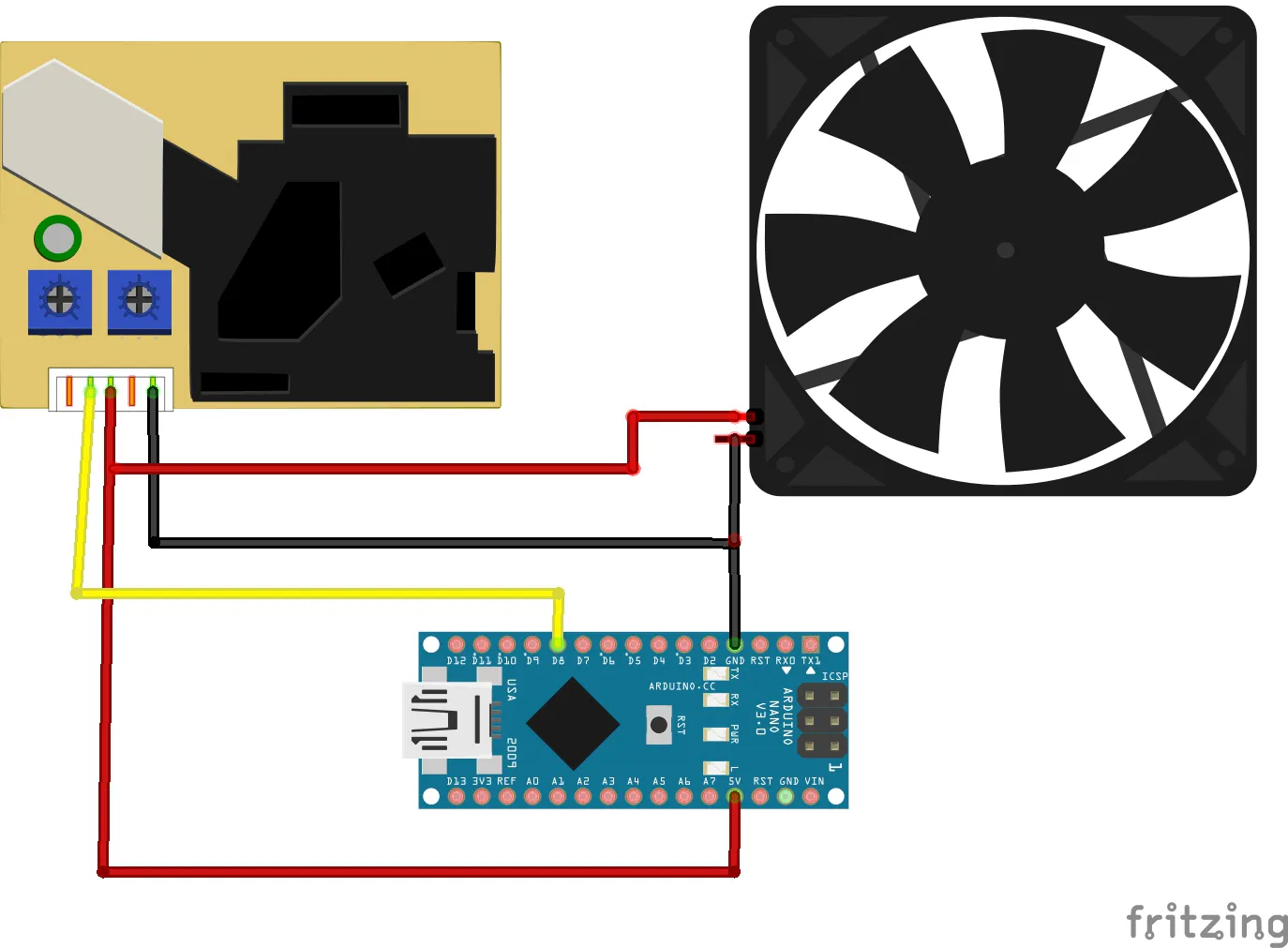# PPD42NS Arduino Air Quality Monitor in 3D Printed Enclosure

Improve reliability of measurements using PPD42NS sensor using modular 3D printed solution which includes exclosure box for sensor.

IntermediateFull instructions provided2,524## Things used in this project

### Hardware componentsArduino Nano R3
×1Seeed Grove - Dust Sensor（PPD42NS）
×1
 Mini fan 5v , 25mm
×1

### Software apps and online servicesArduino IDE

### Hand tools and fabrication machinesSoldering iron (generic)3D Printer (generic)

## Schematics

### Schematic## Code

### Code (serial monitor)

Arduino
```int pin = 8;
unsigned long duration;
unsigned long starttime;
unsigned long sampletime_ms = 3000;//sampe 3s ;
unsigned long lowpulseoccupancy = 0;
float ratio = 0;
float concentration = 0;

void setup()
{
Serial.begin(9600);
pinMode(pin,INPUT);
starttime = millis();//get the current time;
}

void loop()
{
duration = pulseIn(pin, LOW);
lowpulseoccupancy = lowpulseoccupancy+duration;

if ((millis()-starttime) > sampletime_ms)//if the sampel time == 30s
{
ratio = lowpulseoccupancy/(sampletime_ms*10.0);  // Integer percentage 0=>100
concentration = 1.1*pow(ratio,3)-3.8*pow(ratio,2)+520*ratio+0.62; // using spec sheet curve
Serial.print(lowpulseoccupancy);
Serial.print(",");
Serial.print(ratio);
Serial.print(",");
Serial.println(concentration);
lowpulseoccupancy = 0;
starttime = millis();
}
}
```

### Code (serial plotter)

Arduino
```int pin = 8;
unsigned long duration;
unsigned long starttime;
unsigned long sampletime_ms = 500;//sampe 0.5s ;
unsigned long lowpulseoccupancy = 0;
float ratio = 0;
float concentration = 0;

void setup()
{
Serial.begin(9600);
pinMode(pin,INPUT);
starttime = millis();//get the current time;
}

void loop()
{
duration = pulseIn(pin, LOW);
lowpulseoccupancy = lowpulseoccupancy+duration;

if ((millis()-starttime) > sampletime_ms)//if the sampel time == 30s
{
ratio = lowpulseoccupancy/(sampletime_ms*10.0);  // Integer percentage 0=>100
concentration = 1.1*pow(ratio,3)-3.8*pow(ratio,2)+520*ratio+0.62; // using spec sheet curve

Serial.println(ratio);
Serial.print(" ");

lowpulseoccupancy = 0;
starttime = millis();
}
}
```

## Credits

### Mirko Pavleski

8 projects • 253 followers